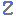Visit Zacobria Webshop

C# code Example of converting RPY/Euler angles to Rotation Vector/Angle Axis for Universal-Robots.

C# code Example of converting RPY/Euler angles to Rotation Vector/Angle Axis for Universal-Robots.

Here’s my C# implementation based on Erwin’s math. I noticed you have to change the theta value depending on the sign of your roll. At least this works for me… please point out any flaws. Thanks.
Given a direction vector, convert that to a roll and a yaw and convert that to a rotation vector:

```/// <summary>
/// Gets the roll pitch and yaw from a direction vector. 0,0,0 is up.
/// </summary>
/// <param name="d">Direction vector. Must have a length of 1</param>
public Vector3 ToRollPitchYaw(Vector3 d)
{
d = Extensions.Normalize(d);
double roll, pitch = 0, yaw = 0;
double pi = Math.PI;
roll = Math.Acos(d.Z);
if (d.X == 0 && d.Y == 0)
{
yaw = 0;
}
else
{
Vector3 noZ = new Vector3 { X = d.X, Y = d.Y, Z = 0 };
noZ = Extensions.Normalize(noZ);
if (d.X <= 0)
yaw = -Math.Acos(-noZ.Y);
else
yaw = Math.Acos(-noZ.Y);
}
if (pitch < -pi)
pitch = 2 * pi + pitch;
else if (pitch > pi)
pitch = pitch - 2 * pi;

Vector3 rpy = new Vector3 { X = (float)roll, Y = (float)pitch, Z = (float)yaw };
return rpy;
}

/// <summary>
/// Converts a roll pitch yaw vector to a rotation vector.
/// </summary>
/// <param name="d">A vector where X=Roll, Y=Pitch, Z=Yaw</param>
/// <returns>A rotation vector with rx, ry and rz used to rotate the TCP of UR10</returns>
public Vector3 ToRotVector(Vector3 rpy)
{
float roll = rpy.X;
float pitch = rpy.Y;
float yaw = rpy.Z;
if (roll == 0 && pitch == 0 && yaw == 0)
return new Vector3 {X=0, Y=0, Z=0};
Matrix3 RollM = new Matrix3();
RollM.M00 = 1; RollM.M01 = 0; RollM.M02 = 0;
RollM.M10 = 0; RollM.M11 = Math.Cos(roll); RollM.M12 = -Math.Sin(roll);
RollM.M20 = 0; RollM.M21 = Math.Sin(roll); RollM.M22 = Math.Cos(roll);

Matrix3 PitchM = new Matrix3();
PitchM.M00 = Math.Cos(pitch); PitchM.M01 = 0; PitchM.M02 = Math.Sin(pitch);
PitchM.M10 = 0; PitchM.M11 = 1; PitchM.M12 = 0;
PitchM.M20 = -Math.Sin(pitch); PitchM.M21 = 0; PitchM.M22 = Math.Cos(pitch);

Matrix3 YawM = new Matrix3();
YawM.M00 = Math.Cos(yaw); YawM.M01 = -Math.Sin(yaw); YawM.M02 = 0;
YawM.M10 = Math.Sin(yaw); YawM.M11 = Math.Cos(yaw); YawM.M12 = 0;
YawM.M20 = 0; YawM.M21 = 0; YawM.M22 = 1;

Matrix3 Rot = new Matrix3();

//rot = yaw * pitch * roll
Rot = Matrix3.Dot(YawM, Matrix3.Dot(PitchM, RollM));

double rotSum = Rot.M00 + Rot.M11 + Rot.M22 - 1;
double alpha = Math.Acos(rotSum / 2);
double theta = 0;
if (roll >= 0)
theta = alpha;
else
theta = 2 * Math.PI - alpha;
double my = 1d / (2 * Math.Sin(theta));

double rx = my * (Rot.M21 - Rot.M12) * theta;
double ry = my * (Rot.M02 - Rot.M20) * theta;
double rz = my * (Rot.M10 - Rot.M01) * theta;

Vector3 rotationVector = new Vector3();
rotationVector.X = (float)rx;
rotationVector.Y = (float)ry;
rotationVector.Z = (float)rz;
return rotationVector;
}

```

Disclaimer: While the Zacobria Pte. Ltd. believes that information and guidance provided is correct, parties must rely upon their skill and judgement when making use of them. Zacobria Pte. Ltd. assumes no liability for loss or damage caused by error or omission, whether such an error or omission is the result of negligence or any other cause. Where reference is made to legislation it is not to be considered as legal advice. Any and all such liability is disclaimed.

If you need specific advice (for example, medical, legal, financial or risk management), please seek a professional who is licensed or knowledgeable in that area.

Author:
By Zacobria Lars Skovsgaard
Accredited 2015-2018 Universal Robots support Centre and Forum.